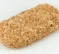## Matlab/Octave loop vectorizationStarted by 3 years ago6 replieslatest reply 3 years ago212 views
My Octave source code:

pkg load signal
clear all;
N=20;
fs=44100;
f=1000;
w=2*pi*f;
t=0:1/fs:1              % 1 second

% RAW square wave
w_sqr = square(w*t);          % RAW Square wave

figure;
plot(t,w_sqr);
axis([0 1 -1.2 1.2]);
grid on;
hold on;
audiowrite('test_square_wave_RAW.wav',w_sqr,fs);

% approximation
for t=0:1/fs:1
for n=1:N
%Fourier-Fejér taper
sum = sum + ((n-2*N-2)*(cos(pi*n)-1)*sin(n*w*t))/(2*pi*n*(N + 1));
end
Ffej(i)=2*sum;
i=i+1;
sum=0;
end;
Ffej = Ffej';
plot(t,Ffej);
axis([0 1 -1.2 1.2]);
audiowrite('test_square_wave_Ffej.wav',Ffej,fs);

figure;
NFFT = 4096;
L=length(w_saw);
X = fft(w_saw,NFFT);
X = X(1:NFFT/2+1);
Px1 = X.*conj(X)/(NFFT*L);
f = fs*(0:NFFT/2)/NFFT;
plot(f,10*log10(Px1),'g', 'linewidth', 3);
hold on;

L=length(Ffej);
X = fft(Ffej,NFFT);
X = X(1:NFFT/2+1);
Px2 = X.*conj(X)/(NFFT*L);
f = fs*(0:NFFT/2)/NFFT;
plot(f,10*log10(Px2),'k','linestyle','--', 'linewidth', 2);
title('Single Sided Power Spectral Density');
xlabel('Frequency (Hz)')
ylabel('Power Spectral Density- P_{xx} dB/Hz');
legend('w_sqr', 'Ffej');
ylim([-60 0]);
grid on;
to approximate square wave is really sloooow... .

I've tried to vectorize code but, (as being a old-school-programmer) can't figure out the vectorization logic ... just get errors. Is the sum calculation vectorizable and how to do it correctly?
[ - ]N=20;
fs=44100;
f=1000;
w=2*pi*f;
t=0:1/fs:1
% approximation
sum_ = sum((((1:N)-2*N-2).*(cos(pi*(1:N))-1).*sin((1:N).*w.*(0:1/fs:1)'))./(2*pi*(1:N)*(N + 1)), 2);
Ffej = 2*sum_;
Ffej = Ffej'; 
[ - ]Thank you very much!

My for-loop code took over one hour to get result (also seemed to use one core only) ... your code gave result immediately.

[ - ]You could probably simplify/speedup the line by doing proper matrix algebra instead of just dot-products and sums, but that would require spending 5 minutes to actually understand what the code does. I'd rather not :-)

[ - ]Just curious why Octave or Matlab tool makers can't automate that job and convert user slow loops to vectors. I have seen some field matlab designs full of slow loops that took hours to finish if it didn't break down.

[ - ]